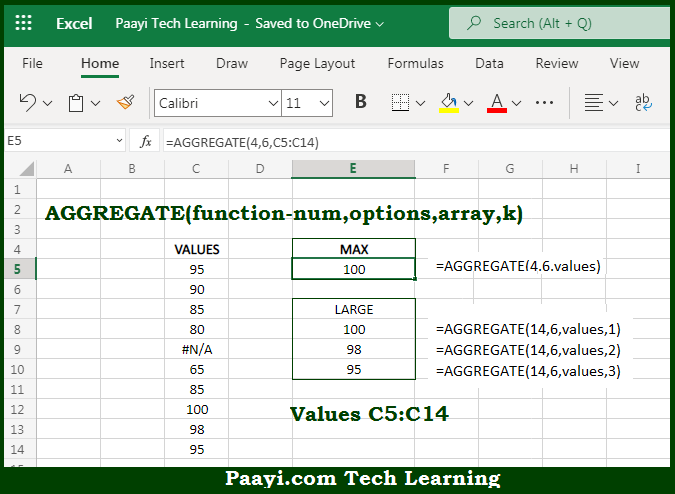# Learn How to Use Microsoft Excel AGGREGATE Function

Written by | 0 Comments | 560 Views

In this article, you will learn how to use the Microsoft Excel AGGREGATE function and its prime function in Microsoft Excel. You will also get to know the Microsoft Excel AGGREGATE function return value and syntax with the help of some examples.

Microsoft Excel AGGREGATE Function

The main function of the Microsoft Excel AGGREGATE function is to return the Aggregate calculation that implies - Average, max, Count, etc., and sometimes the operation ignores the hidden rows and errors. You can perform nineteen operations using the Aggregate function which is available, and specified by the first argument is given below. So, with the help of the Microsoft Excel AGGREGATE function, you can easily return the Aggregate calculation.

Return Value of AGGREGATE Function

The return value depends on the function specified.

Syntax of AGGREGATE Function

=AGGREGATE(function-number, options, ref1, ref2)

Where the arguments:

function-number: This is the operation to perform (1-19).

options: There are the values to ignore (0-7).

ref1: First argument.

ref2: Second argument (k).

How to Use Microsoft Excel AGGREGATE Function?Microsoft Excel AGGREGATE function is to return the Aggregate calculation that implies - Average, max, Count, etc., and sometimes the operation ignores the hidden rows and errors. You can perform nineteen operations using the Aggregate function which is available, and specified by the first argument. So, with the help of the Microsoft Excel AGGREGATE function, you can easily return the aggregate of calculation, with the help of 19 operations available, and the operations performed are specified as the number, which appears as the first argument in the function. The second argument, options, controls how AGGREGATE handles errors and values in hidden rows. We have provided the table below for all available options.

Function numbers

The table here lists the function numbers available with the AGGREGATE function, along with the name of the related function. The third column, Ref2, indicates the second argument value expected when required.

 Function Function Ref2 1 AVERAGE 2 COUNT 3 COUNTA 4 MAX 5 MIN 6 PRODUCT 7 STDEV.S 8 STDEC.P 9 SUM 10 VAR.S 11 VAR.P 12 MEDIAN 13 MODESNGL 14 LARGE k 15 SMALL k 16 PERCENTILE.INC k 17 QUARTILE.INC Quart 18 PERCENTILE.EXC k 19 QUARTILE.EXC Quart

...